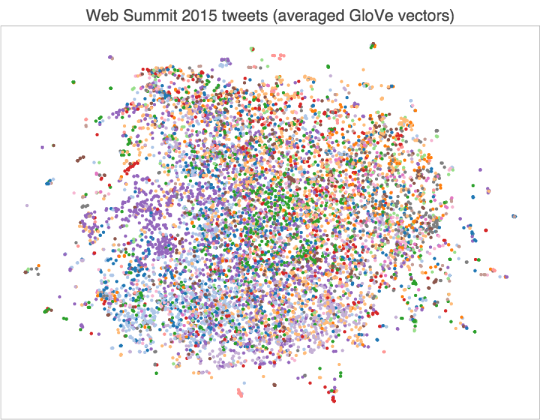### 代码在 https://github.com/crownpku/text2vec## 安装

``````git clone https://github.com/crownpku/text2vec
``````

``````python -m spacy download en
``````

## 文本向量化

• Term Frequency x Inverse Document Frequency, Tf-Idf

• Latent Semantic Indexing, LSI (or sometimes LSA)

• Random Projections, RP

• Latent Dirichlet Allocation, LDA

• Hierarchical Dirichlet Process, HDP

``````nlp  = spacy.load('en')
def _document_vector(self, doc, docs_dict, nlp):
doc_vector = [nlp(word).vector for word in doc if word in docs_dict.token2id]
return np.mean(doc_vector, axis=0)
``````

``````def tfidf_weighted_wv(self):
#tf-idf
docs_vecs   = self._get_tfidf(self.docs, self.docs_dict)

#Load glove embedding vector for each TF-IDF term
tfidf_emb_vecs = np.vstack([self.nlp(self.docs_dict[i]).vector for i in range(len(self.docs_dict))])

#To get a TF-IDF weighted Glove vector summary of each document,
#we just need to matrix multiply docs_vecs with tfidf_emb_vecs
docs_emb = np.dot(docs_vecs, tfidf_emb_vecs)

return docs_emb
``````

``````import text2vec

t2v = text2vec.text2vec(doc_list)

# Use TFIDF
docs_tfidf = t2v.get_tfidf()

# Use Latent Semantic Indexing(LSI)
docs_lsi = t2v.get_lsi()

# Use Random Projections(RP)
docs_rp = t2v.get_rp()

# Use Latent Dirichlet Allocation(LDA)
docs_lda = t2v.get_lda()

# Use Hierarchical Dirichlet Process(HDP)
docs_hdp = t2v.get_hdp()

# Use Average of Word Embeddings
docs_avgw2v = t2v.avg_wv()

# Use Weighted Word Embeddings wrt. TFIDF
docs_emb = t2v.tfidf_weighted_wv()
``````

## 文本相似度/距离

• Cosine Similarity

• Euclidean Distance

• Triangle’s Area Similarity (TS)

• Sector’s Area Similairity (SS)

• TS-SS

text2vec项目中提供了`text2vec.simical`的方法，可以很方便计算向量间不同种类的相似度/距离。

``````# Initialize
import text2vec
sc = text2vec.simical(docs_emb, docs_emb)

# Use Cosine
simi_cos = sc.Cosine()

# Use Euclidean
simi_euc = sc.Euclidean()

# Use Triangle's Area Similarity (TS)
simi_ts = sc.Triangle()

# Use Sector's Area Similairity (SS)
simi_ss = sc.Sector()

# Use TS-SS
simi_ts_ss = sc.TS_SS()
``````

## 未来工作

• 一个显而易见的事情是把`text2vec.text2vec`推广到中文文本向量化。这里需要做的是将spacy的模型读取替换成中文word embedding的读取，同时要留意中文tokenize的这一步。

• 现在的`text2vec.simical`是计算两个向量的相似度。未来可以在这个基础上开发一个多线程的相似度匹配的方法，方便进行聚类或者寻找最相似文档的任务，在chatbot问题匹配或是无标注数据的非监督任务中会有用处。

• 可以包装进SVM等几个常用分类器，来对有标签的标注数据进行监督学习的文本分类。

• 现在的方式，用户可以对`text2vec.text2vec`提供的向量化方法结果进行组合叠加产生新的文本向量；未来期望加入更多的更创新的短文本向量化的方法，如已经实现的词向量的TF-IDF加权平均的方法。